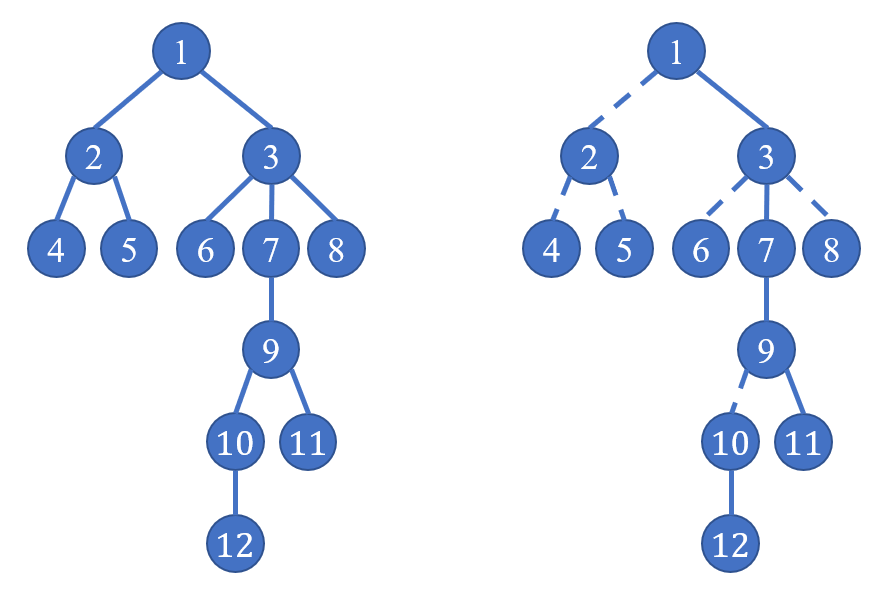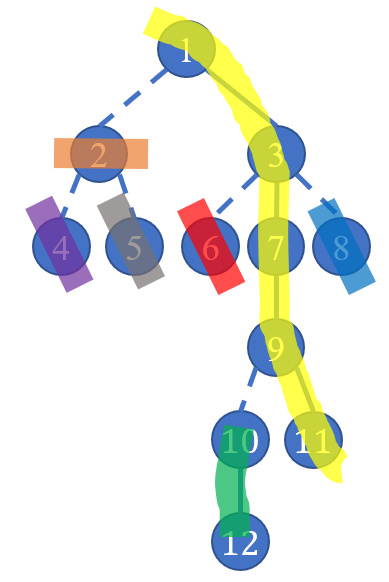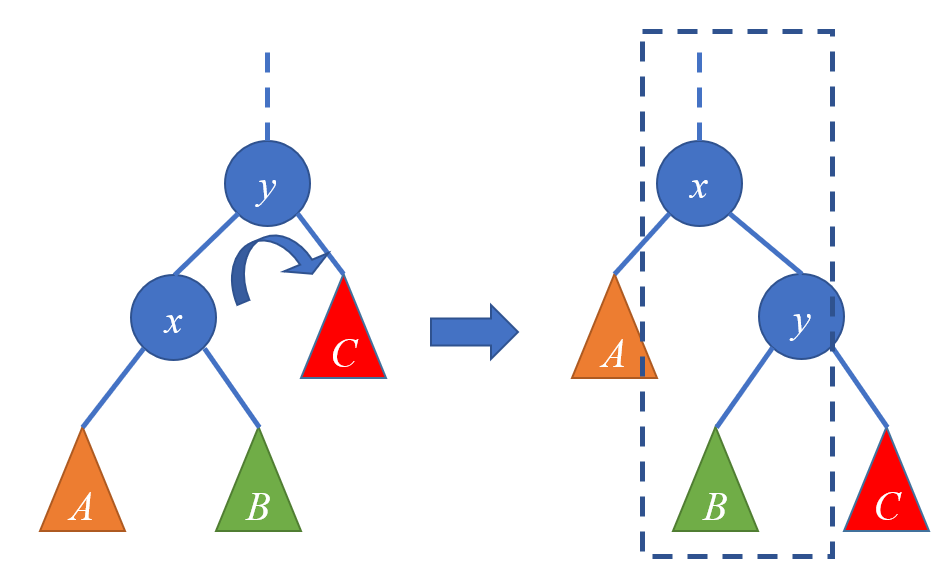## 链剖

### 实链剖分## LCT

LCT叫做 Link-cut Tree ，顾名思义就是可以连边和断边的树。不过它可不是一般的树，它支持在$O(\log n)$的时间内查询树上任意两点间最短路径上的信息。

### Rotate(x)### splay(x)

LCT需要翻转平衡树，因此在splay操作之前，先回溯到根，然后从上到下依次pushdown()以保证树的形态的 正确性。

### access(x)

splay可以维护一条实链上的全部信息。然而如果我们需要的信息不在一条实链上怎么办呢？

access(x)可以把从$x$到根的路径上的边全部变为实边，而把$x$与其儿子的边全部变为虚边。

1. 首先操作$x$，$x$一定在它所属的splay中，让$x$转到splay的根，接下来断掉$x$的右儿子。此时$x$所在的splay是以$x$为最深点的实链。接下来处理$x$的父亲，由于$x$已经是splay的根了，这里的父亲就指的是另一棵splay中的点，也就是当前$x$所在实链的顶端节点在原图上的父亲。参考上图，在黄色链中若$12$为根，则$12$的父亲是$9$，也就是$10$在原图上的父亲。定义$x$所在的splay为$A$，$x$的父亲为$y$，$y$所在的splay为$B$。
2. 把$y$旋转到它所在splay的根，而此时实链的方向要切换到$x$，原来的就不能保留。因为$y$的深度比$A$中任何一个节点都要大，所以直接把$y$的右儿子置为$x$即可。原来的实链就自动变虚了。
3. 然后递归$y$的父亲，重复第$2$步，直到$y$为根。（注意不要把splay的根和树的根弄混了。

### getroot(x)

access(x)，使$x$和根连起来，然后splay(x)，方便访问平衡树。接着就很容易找到平衡树中的最小元素了。

## Code:

#include<cstdio>
#include<cstring>
#define ls ch[k]
#define rs ch[k]
#define which(k) (ch[fa[k]]==k)
#define isroot(k) (ch[fa[k]]!=k&&ch[fa[k]]!=k)
//树的形态一旦改变 必要情况下要maintain
int key,ch,lazy;
int sum,fa;
void pushdown(int k)
{
if(lazy[k])
{
lazy[k]=0;
lazy[ls]^=1;
lazy[rs]^=1;
int tmp=ls;
ls=rs;
rs=tmp;
}
}
void maintain(int k)
{
sum[k]=sum[ls]^key[k]^sum[rs];
}
void Rotate(int k)
{
int y=fa[k];
if(!isroot(y))//调用Rotate(k)时就保证了k不是根
ch[which(y)][fa[y]]=k;
bool d=which(k);

fa[k]=fa[y];//第一步 二者互相换
fa[y]=k;
ch[d][y]=ch[!d][k];//第二步 只换一个儿子
fa[ch[d][y]]=y;//更新这个儿子
ch[!d][k]=y;//第三步 重新认儿子
maintain(y);
maintain(k);//第四步更新 （不更新父亲）
}
int stk;
void splay(int k)//把k旋到当前树的根
{
int x=k;
int tp=0;
while(!isroot(x))
{
stk[++tp]=x;
x=fa[x];
}
stk[++tp]=x;
while(tp)
pushdown(stk[tp--]);//把可能影响到的位置全部下放

while(!isroot(k))
{
int y=fa[k];
if(!isroot(y))
Rotate(which(k)^which(y)?k:y);
Rotate(k);
}
}
void access(int k)//把k与根连起来
{
for(int x=k,y=0;x;y=x,x=fa[x])
{
splay(x);
ch[x]=y;//理解
maintain(x);
}
}
void makeroot(int k)//使k成为根
{
access(k);
splay(k);
lazy[k]^=1;
}
void split(int x,int y)
{
makeroot(x);
access(y);
splay(y);
}
int getroot(int k)
{
access(k);
splay(k);
while(ls)
k=ls;
return k;
}
{
makeroot(x);
if(getroot(y)==x)
return;
fa[x]=y;
}
void cut(int x,int y)
{
makeroot(x);
if(getroot(y)!=x||fa[x]!=y||ch[x])//x有右儿子也不行
return;
fa[x]=0;
ch[y]=0;
maintain(y);
}
int main()
{
int n,m,op,u,v;
scanf("%d%d",&n,&m);
for(int i=1;i<=n;++i)
scanf("%d",&key[i]);
for(int i=1;i<=m;++i)
{
scanf("%d%d%d",&op,&u,&v);
if(op==0)
{
split(u,v);
printf("%d\n",sum[v]);
}
else if(op==1)
else if(op==2)
cut(u,v);
else
{
access(u);
splay(u);
key[u]=v;
maintain(u);
}
}
return 0;
}


### 1 说点什么0 Followers

Most reacted commentRecent comment authorswpDiscuz